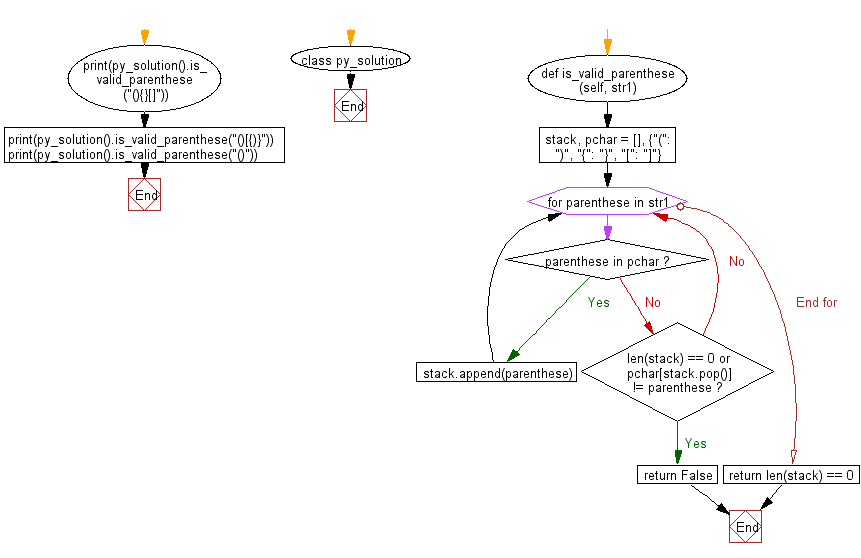﻿ Python: Validity of a string of parentheses - w3resource# Python: Validity of a string of parentheses

## Python Class: Exercise-3 with Solution

Write a Python class to find validity of a string of parentheses, '(', ')', '{', '}', '[' and ']. These brackets must be close in the correct order,
for example "()" and "()[]{}" are valid but "[)", "({[)]" and "{{{" are invalid.

Sample Solution:

Python Code:

``````class py_solution:
def is_valid_parenthese(self, str1):
stack, pchar = [], {"(": ")", "{": "}", "[": "]"}
for parenthese in str1:
if parenthese in pchar:
stack.append(parenthese)
elif len(stack) == 0 or pchar[stack.pop()] != parenthese:
return False
return len(stack) == 0

print(py_solution().is_valid_parenthese("(){}[]"))
print(py_solution().is_valid_parenthese("()[{)}"))
print(py_solution().is_valid_parenthese("()"))
```
```

Sample Output:

```True
False
True
```

Pictorial Presentation:Flowchart:## Visualize Python code execution:

The following tool visualize what the computer is doing step-by-step as it executes the said program:

Python Code Editor:

What is the difficulty level of this exercise?

Test your Programming skills with w3resource's quiz.

﻿

## Python: Tips of the Day

The Zip() Function:

```>>> students = ('John', 'Mary', 'Mike')
>>> ages = (15, 17, 16)
>>> scores = (90, 88, 82, 17, 14)
>>> for student, age, score in zip(students, ages, scores):
...     print(f'{student}, age: {age}, score: {score}')
...
John, age: 15, score: 90
Mary, age: 17, score: 88
Mike, age: 16, score: 82
>>> zipped = zip(students, ages, scores)
>>> a, b, c = zip(*zipped)
>>> print(b)
(15, 17, 16)
```